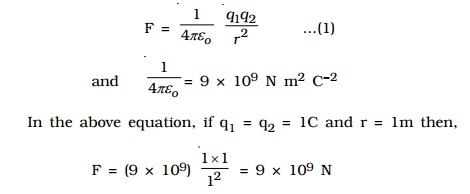Home | | Physics | Electrostatics - frictional electricity

# Electrostatics - frictional electricityThe substances which acquire charges on rubbing are said to be 'electrified' or charged. These terms are derived from the Greek word elektron, meaning amber. The electricity produced by friction is called frictional electricity. If the charges in a body do not move, then, the frictional electricity is also known as Static Electricity.

Electrostatics

Electrostatics is the branch of Physics, which deals with static electric charges or charges at rest. In this chapter, we shall study the basic phenomena about static electric charges. The charges in a electrostatic field are analogous to masses in a gravitational field. These charges have forces acting on them and hence possess potential energy. The ideas are widely used in many branches of electricity and in the theory of atom.

Electrostatics - frictional electricity

In 600 B.C., Thales, a Greek Philosopher observed that, when a piece of amber is rubbed with fur, it acquires the property of attracting light objects like bits of paper. In the 17th century, William Gilbert discovered that, glass, ebonite etc, also exhibit this property, when rubbed with suitable materials.

The substances which acquire charges on rubbing are said to be 'electrified' or charged. These terms are derived from the Greek word elektron, meaning amber. The electricity produced by friction is called frictional electricity. If the charges in a body do not move, then, the frictional electricity is also known as Static Electricity.

1.  Two kinds of charges

1.    If a glass rod is rubbed with a silk cloth, it acquires positive charge while the silk cloth acquires an equal amount of negative charge.

2.    If an ebonite rod is rubbed with fur, it becomes negatively charged, while the fur acquires equal amount of positive charge. This classification of positive and negative charges were termed by American scientist, Benjamin Franklin.

Thus, charging a rod by rubbing does not create electricity, but simply transfers or redistributes the charges in a material.

2. Like charges repel and unlike charges attract each other - experimental verification.

A charged glass rod is suspended by a silk thread, such that it swings horizontally. Now another charged glass rod is brought near the end of the suspended glass rod. It is found that the ends of the two rods repel each other as diagram 1. However, if a charged ebonite rod is brought near the end of the suspended rod, the two rods attract each other as diagram 2. The above experiment shows that like charges repel and unlike charges attract each other.The property of attraction and repulsion between charged bodies have many applications such as electrostatic paint spraying, powder coating, fly?ash collection in chimneys, ink-jet printing and photostat copying (Xerox) etc.

3. Conductors and Insulators

According to the electrostatic behaviour, materials are divided into two categories : conductors and insulators (dielectrics). Bodies which allow the charges to pass through are called conductors. e.g. metals, human body, Earth etc. Bodies which do not allow the charges to pass through are called insulators. e.g. glass, mica, ebonite, plastic etc.

4. Basic properties of electric charge

Quantisation of electric charge

The  fundamental  unit  of  electric  charge  (e)  is  the  charge carried by the electron and its unit is coulomb. e has the magnitude 1.6 x 10-19 C.

In nature, the electric charge of any system is always an integral multiple of the least amount of charge. It means that the quantity can take only one of the discrete set of values. The charge, q = ne where n is an integer.

Conservation of electric charge

Electric charges can neither be created nor destroyed. According to the law of conservation of electric charge, the total charge in an isolated system always remains constant. But the charges can be transferred from one part of the system to another, such that the total charge always remains conserved. For example, Uranium (92U238) can decay by emitting an alpha particle (2He4 nucleus) and transforming to thorium (90Th234).

92U238 --- > 90Th234 + 2He4

Total charge before decay = +92e, total charge after decay = 90e + 2e. Hence, the total charge is conserved. i.e. it remains constant.

The total electric charge of a system is equal to the algebraic sum of electric charges located in the system. For example, if two charged bodies of charges +2q, -5q are brought in contact, the total charge of the system is -3q.

5. Coulomb's law

The force between two charged bodies was studied by Coulomb in 1785.

Coulomb's law states that the force of attraction or repulsion between two point charges is directly proportional to the product of the charges and inversely proportional to the square of the distance between them. The direction of forces is along the line joining the two point charges.

Let q1 and q2 be two point charges placed in air or vacuum at a distance r apart . Then, according to Coulomb's law,where k is a constant of proportionality. In air or vacuum,

k = 1/4??o , where ?o is the permittivity of free space (i.e., vacuum) and the value of ?o is 8.854 x 10?12 C2 N?1 m?2.One Coulomb is defined as the quantity of charge, which when placed at a distance of 1 metre in air or vacuum from an equal and similar charge, experiences a repulsive force of 9 x 109 N.

If the charges are situated in a medium of permittivity ?, then the magnitude of the force between them will be,Coulombxs law x vector formSo, the forces exerted by charges on each other are equal in magnitude and opposite in direction.

6. Principle of Superposition

The principle of superposition is to calculate the electric force experienced by a charge q1 due to other charges q2, q3 ..... qn.

The total force on a given charge is the vector sum of the forces exerted on it due to all other charges.Study Material, Lecturing Notes, Assignment, Reference, Wiki description explanation, brief detail
Physics : Higher Secondary School : Electrostatics - frictional electricity |